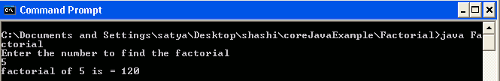# Write a program to find a factorial in any given number

This programming tutorial will teach you how to write a factorial of any given number.## Write a program to find a factorial in any given number

This programming tutorial will teach you how to write a factorial of any given number. Factorial of a non-negative integer is denoted by n!, which is product of all positive number less than or equal to n. For example

4! = 4 * 3 *2 * 1 that is equal to 24, and factorial of  0! is 0 always.

Example : A program to find the factorial of any given number

```  import java.util.Scanner;
class Factorial
{
public static void main(String args[])
{
int fact=1;
System.out.println("Enter the number to find the factorial");
Scanner sin=new Scanner(System.in);
int f=sin.nextInt();
for(int i=1;i<=f;i++)
{
fact=fact*i;
}
System.out.println("factorial of "+f+" is = " +fact);
}
}```

Description : In the above example suppose user entered 5, then 5 is stored in scanner class object and using for loop, iterating  the loop from 1 to 5(i<=f) until it reaches 5 multiplying each value of i with fact value i.e. 1 and storing it in fact variable. Loop will iterate five times ( 1=1*1, 2=1*2 ......) and stored in fact variable, when the value of i become 5 control come out from the loop and print the value of fact.

Output : Compiling and executing the above program.